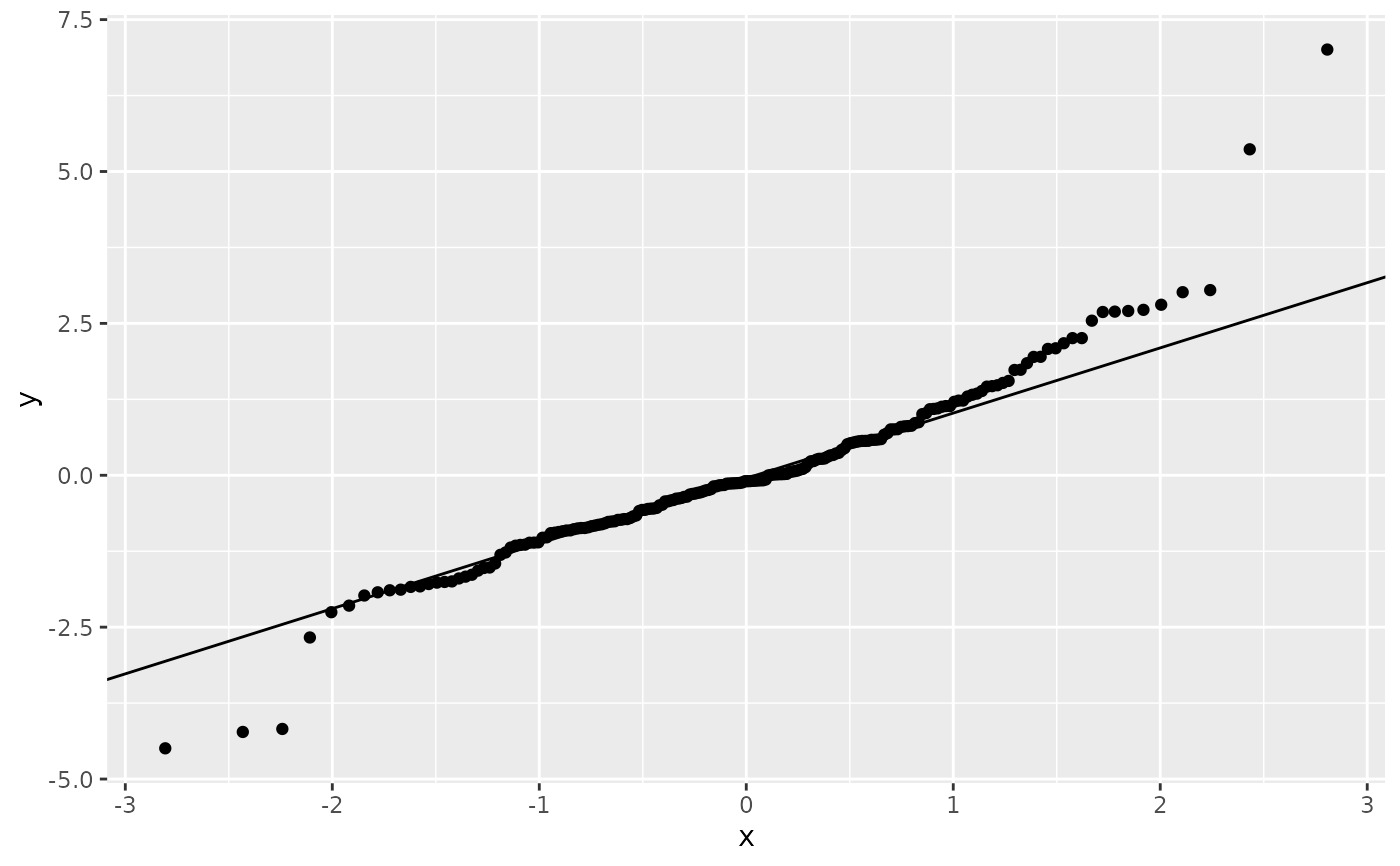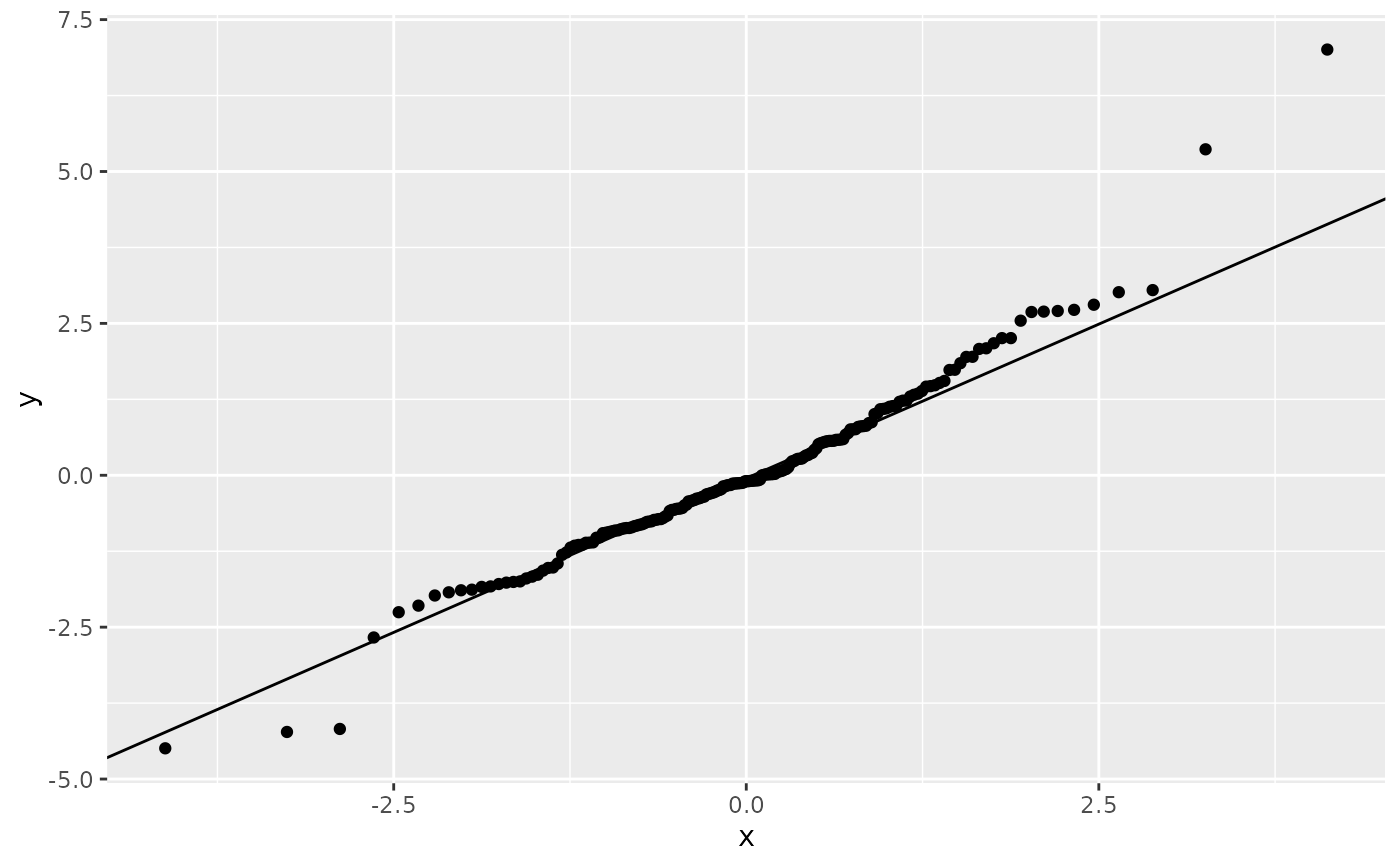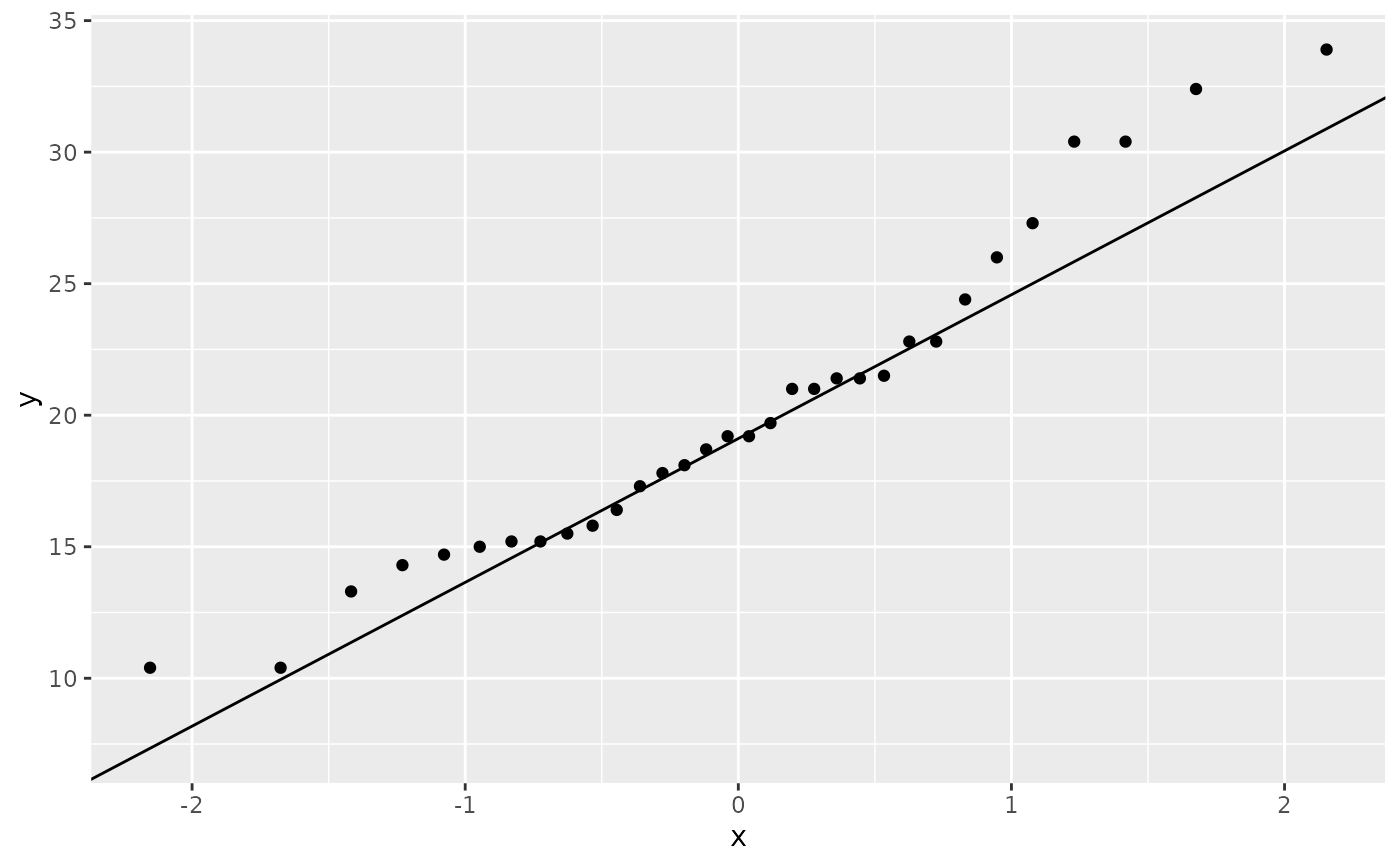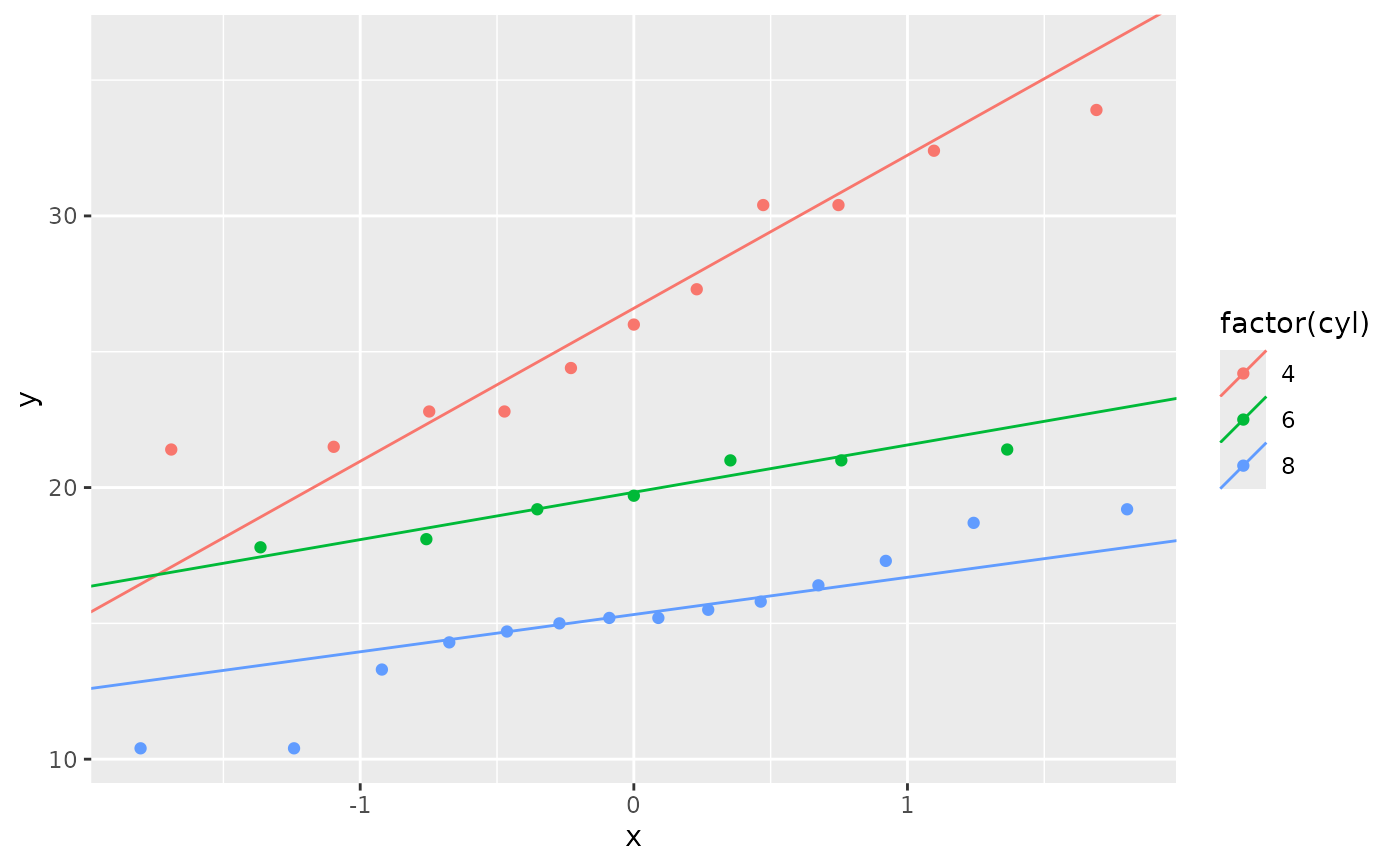geom_qq() and stat_qq() produce quantile-quantile plots. geom_qq_line() and stat_qq_line() compute the slope and intercept of the line connecting the points at specified quartiles of the theoretical and sample distributions.

## Usage

geom_qq_line(
mapping = NULL,
data = NULL,
geom = "path",
position = "identity",
...,
distribution = stats::qnorm,
dparams = list(),
line.p = c(0.25, 0.75),
fullrange = FALSE,
na.rm = FALSE,
show.legend = NA,
inherit.aes = TRUE
)

stat_qq_line(
mapping = NULL,
data = NULL,
geom = "path",
position = "identity",
...,
distribution = stats::qnorm,
dparams = list(),
line.p = c(0.25, 0.75),
fullrange = FALSE,
na.rm = FALSE,
show.legend = NA,
inherit.aes = TRUE
)

geom_qq(
mapping = NULL,
data = NULL,
geom = "point",
position = "identity",
...,
distribution = stats::qnorm,
dparams = list(),
na.rm = FALSE,
show.legend = NA,
inherit.aes = TRUE
)

stat_qq(
mapping = NULL,
data = NULL,
geom = "point",
position = "identity",
...,
distribution = stats::qnorm,
dparams = list(),
na.rm = FALSE,
show.legend = NA,
inherit.aes = TRUE
)

## Arguments

mapping

Set of aesthetic mappings created by aes(). If specified and inherit.aes = TRUE (the default), it is combined with the default mapping at the top level of the plot. You must supply mapping if there is no plot mapping.

data

The data to be displayed in this layer. There are three options:

If NULL, the default, the data is inherited from the plot data as specified in the call to ggplot().

A data.frame, or other object, will override the plot data. All objects will be fortified to produce a data frame. See fortify() for which variables will be created.

A function will be called with a single argument, the plot data. The return value must be a data.frame, and will be used as the layer data. A function can be created from a formula (e.g. ~ head(.x, 10)).

geom

The geometric object to use to display the data, either as a ggproto Geom subclass or as a string naming the geom stripped of the geom_ prefix (e.g. "point" rather than "geom_point")

position

Position adjustment, either as a string naming the adjustment (e.g. "jitter" to use position_jitter), or the result of a call to a position adjustment function. Use the latter if you need to change the settings of the adjustment.

...

Other arguments passed on to layer(). These are often aesthetics, used to set an aesthetic to a fixed value, like colour = "red" or size = 3. They may also be parameters to the paired geom/stat.

distribution

Distribution function to use, if x not specified

dparams

Additional parameters passed on to distribution function.

line.p

Vector of quantiles to use when fitting the Q-Q line, defaults defaults to c(.25, .75).

fullrange

Should the q-q line span the full range of the plot, or just the data

na.rm

If FALSE, the default, missing values are removed with a warning. If TRUE, missing values are silently removed.

show.legend

logical. Should this layer be included in the legends? NA, the default, includes if any aesthetics are mapped. FALSE never includes, and TRUE always includes. It can also be a named logical vector to finely select the aesthetics to display.

inherit.aes

If FALSE, overrides the default aesthetics, rather than combining with them. This is most useful for helper functions that define both data and aesthetics and shouldn't inherit behaviour from the default plot specification, e.g. borders().

## Aesthetics

stat_qq() understands the following aesthetics (required aesthetics are in bold):

• sample

• group

• x

• y

Learn more about setting these aesthetics in vignette("ggplot2-specs").

stat_qq_line() understands the following aesthetics (required aesthetics are in bold):

• sample

• group

• x

• y

Learn more about setting these aesthetics in vignette("ggplot2-specs").

## Computed variables

These are calculated by the 'stat' part of layers and can be accessed with delayed evaluation.
Variables computed by stat_qq():

• after_stat(sample)
Sample quantiles.

• after_stat(theoretical)
Theoretical quantiles.

Variables computed by stat_qq_line():

• after_stat(x)
x-coordinates of the endpoints of the line segment connecting the points at the chosen quantiles of the theoretical and the sample distributions.

• after_stat(y)
y-coordinates of the endpoints.

## Examples

# \donttest{
df <- data.frame(y = rt(200, df = 5))
p <- ggplot(df, aes(sample = y))
p + stat_qq() + stat_qq_line()# Use fitdistr from MASS to estimate distribution params
params <- as.list(MASS::fitdistr(df$y, "t")$estimate)
#> Warning: NaNs produced
#> Warning: NaNs produced
#> Warning: NaNs produced
ggplot(df, aes(sample = y)) +
stat_qq(distribution = qt, dparams = params["df"]) +
stat_qq_line(distribution = qt, dparams = params["df"])# Using to explore the distribution of a variable
ggplot(mtcars, aes(sample = mpg)) +
stat_qq() +
stat_qq_line()ggplot(mtcars, aes(sample = mpg, colour = factor(cyl))) +
stat_qq() +
stat_qq_line()# }